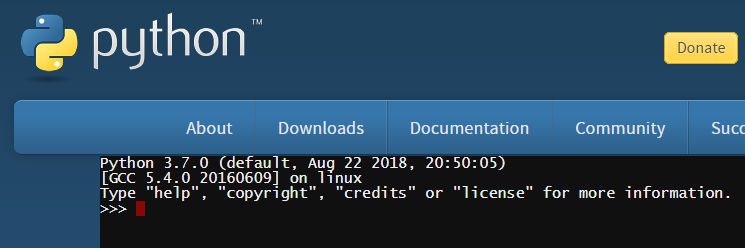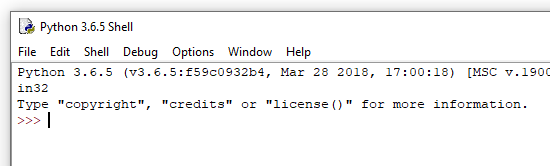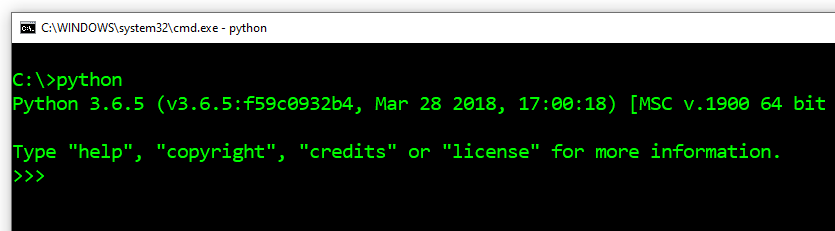# Obeleži sve kategorije koje odgovaraju problemu

### Još detalja - opišite nam problem

Uspešno ste prijavili problem!
Status problema i sve dodatne informacije možete pratiti klikom na link.
Nažalost nismo trenutno u mogućnosti da obradimo vaš zahtev.
Molimo vas da pokušate kasnije.

# Introduction to Python¶

Python is a very popular general-purpose programming language. It became known for its simplicity, ease of learning and fast programming. Many professional developers use Python at least as an auxiliary language, because they quickly and easily automate various jobs with it. Due to the aforementioned good qualities, there are more and more users of Python among people from other professions who use programming in various fields. The Python programming language is free to use, and a large community has been formed around it, contributing to its further development and general support on the Internet.

Python’s programming language makes it clear that programming is not just for professional programmers, the same way as writting is not just for professional writers. There are more and more different jobs that can become somewhat easier, more successful or more productive with moderate programming skills. Therefore, this handbook is not only for future programming professionals, but for anyone who can benefit from programming in their jobs (or elsewhere).

So, let’s look at the basics of Python and see how commands and programs are written in this programming language.

## Python interpreter¶

To execute programs we write on Python, we need a program called Python interpreter. This program interprets and then executes Python commands. Python interpreters can accept whole programs and execute them, and they can also work in interactive mode, in which every command we enter is executed immediately.

The environment in which the Python interpreter executes is called a shell. There are various shells in which the corresponding Python interpreter can be executed. Therefore, we have several ways to launch the Python shell.

Shells online

Website https://www.python.org/shell contains an online shell, which you can use right away for interactive work (it is enough to have internet access).Python installation and IDLE environment

To learn Python programming, it is certainly useful to download Python from https://www.python.org/downloads/ and install it if it is not already installed on your computer. With Python installation, you also get a program called IDLE (integrated development and learning environment). This integrated environment also includes a shell where you can execute Python programs. When you run IDLE on your computer, you get the following window, in which you can work interactively and write and execute Python programs.Shell in command window

Another way to start a Python shell is to open a command window (on Windows systems this is done by running the program cmd) and then type Python in the command window (here we asume that Python is installed so that it is accessible from every folder, otherwise you should first position yourself in the Python interpreter folder).You can choose any shell you like, they are all used the same way.

## Interactive work¶

Start your Python shell. The >>> characters you see represent a prompt. This way the Python interpreter tells us that it is ready to receive the command.

When working interactively, Python interpreter can also be used as a calculator - type an expression and get its value:

>>> 3 + 2
5
>>> 3 - 2
1
>>> 4 * 2
8
>>> 4 / 2
2.0


The following symbols are used for basic calculating operations in Python (as in most programming languages):

• addition: +

• subtraction: -

• multiplication: *

• division: /

In addition to these basic and most commonly used ones, we sometimes need a few other operations, which are used less frequently. Those are:

• integer division (taking the whole part of the quotient): //, for example the value of the expression $$7 // 2$$ si $$3$$.

• remainder of a division: %, for example the value of the expression $$7 \% 2$$ is $$1$$.

• the power operator: **, for example the value of the expression $$2 ** 4$$ is $$2^4 = 16$$.

>>> 7 // 2
3
>>> 7 % 2
1
>>> 2 ** 4
16


Computers distinguish between integers and real numbers, write them differently in their memory, and do computations with them in different ways. So in programming, value 2.0 is not quite the same as 2, although the values are mathematically equal (the sign == is used to compare the two values).

>>> 2.0 == 2
True
>>> type(2.0)
<class 'float'>
>>> type(2)
<class 'int'>


What we see means that the first number is real and the second is integer (the word float denotes real numbers, and int denostes integers).

In this regard, note that in Python, the result of the ordinary division / is always a real number, even when the operands are integers and are divisible (there is no remainder). When we want the result of the division between two integers to be an integer, we should use the integer division operator //.

>>> 6/2
3.0
>>> 6//2
3


For the other operations specified, the result is an integer when both operands (the numbers to which the operation applies) are integers, and real if at least one operand is real.

>>> 3 + 2
5
>>> 3.0 + 2
5.0
>>> 3 + 2.0
5.0
>>> 2.0 ** 4
16.0


The rules for calculating expression values are the same as in mathematics:

• The power is computed before the other operations. If there are multiple sequencing power operations, they are performed from right to left.

• Multiplication, division and remainder operations are applied before addition and subtraction. When there are more in a row, they are executed from left to right.

• When we need a different order of computation, we use parentheses (the part in parentheses is calculated first).

>>> (5-3) * (2+2)
8
>>>


We end our work in the Python shell by typing the command quit().

>>> quit()


Make sure you understand the rules of calculating in Python by answering the following questions.

Q-1: What is the value of the expression 5 + 5 * 5?

• 15
• 30
• Correct!
• 50
• Note also the priority of operations (the same as in mathematics).
• 125

Q-2: What is the value of the expression 4 + 11 % 5?

• 3
• 0
• Note also the priority of operations (the same as in mathematics).
• 5
• Correct!
• 6
• The % sign represents reminder operation.

Q-3: What is the value of the expression 10 ** 2 ** 3?

• 60
• symbols ** represent power operator
• 100000000
• Correct!
• 1000000
• Power operators are performed from right to left, so that a ** b ** c is the same as a ** (b ** c).
• 300
• Both specified operators are power operators.

Q-4: What is the value of the expression 15 - 10 // 3?

• 1.666666
• Read again about the symbols and the order of execution (priority) of operations
• 1
• The integer division is performed first
• 11.666666
• The // symbol represents an integer division.
• 12
• Correct!

Q-5: What is the value of the expression 15 / (5 - 2)?

• 5.0
• Correct!
• 5
• The result of this division is always a real number.
• 1.0
• First the part in the parenthesis is calculated.
• 1
• First the part in the parenthesis is calculated.